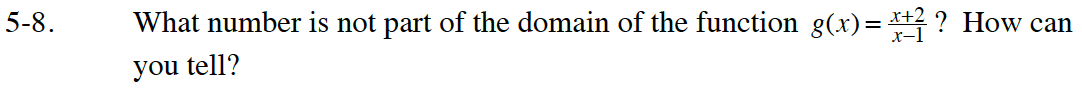### Home > GB8I > Chapter 5 Unit 6 > Lesson INT1: 5.1.1 > Problem5-8

5-8.

What number is not part of the domain of the function g(x) = $\frac { x + 2 } { x - 1 }$? How can you tell? Homework Help ✎It is impossible to divide by zero. What number makes the denominator zero?